Checkout NEET 2022 Question Paper Analysis : Checkout NEET 2022 Question Paper Analysis :

# Exclusive OR gate

The Exclusive-OR gate or XOR gate is achieved by combining standard logic gates together. XOR gate is used extensively in error detection circuits, computational logic comparators and arithmetic logic circuits. The Exclusive OR gate gives an output only if its two inputs are dissimilar, namely if one of them is high (one) and the other is low (zero).

## Symbol and Truth Table of XOR gate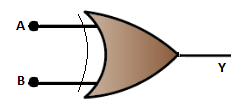### The Truth Table of 2 input XOR gate

The Boolean expression representing the 2 input XOR gate is written as

$$\begin{array}{l}Y=(A\bigoplus B)=\bar{A}.B +A.\bar{B}\end{array}$$
 A B Y 0 0 0 0 1 1 1 0 1 1 1 0

### The Truth Table for 3 input XOR gate

The Boolean expression for the three-input logic gate is given by

$$\begin{array}{l}Y=A\bigoplus B\bigoplus C=A\bar{BC}+\bar{A}B\bar{C}+\bar{AB}C+ABC\end{array}$$
 A B C Y 0 0 0 0 0 0 1 1 0 1 0 1 0 1 1 0 1 0 0 1 1 0 1 0 1 1 0 0 1 1 1 1

## XOR gate equivalent circuit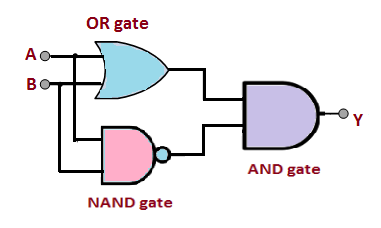In the above figure, three different logic gates are used to form an XOR, this is one of the main disadvantages. One of the easier ways to produce the XOR gate is to use only the combination of the NAND gate or NOR gate

## Construction of XOR gate using NAND gate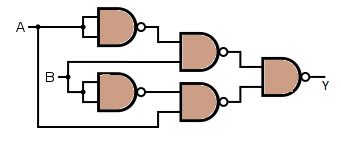## Construction of XOR gate using NOR gate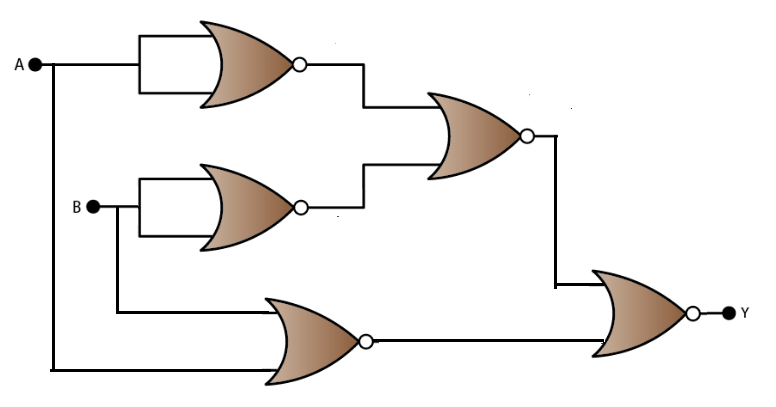## Application of XOR gate

XOR gates are used in circuits that perform arithmetic operations and calculations, especially in half-adders and adders. The ability of the XOR gate to compare two logic levels and give an output dependent upon the input condition is very useful in many computational circuits. Though it is not a basic logic gate, its versatility and usefulness have made it into a standard logic gate. A suitable XOR gate can be used as a pseudo-random number generator.

### Recommended Video:

Current Electricity Class 12 Physics One Shot & Mind Maps | Physics Ch 3 Class 12 | NEET 2022 Exam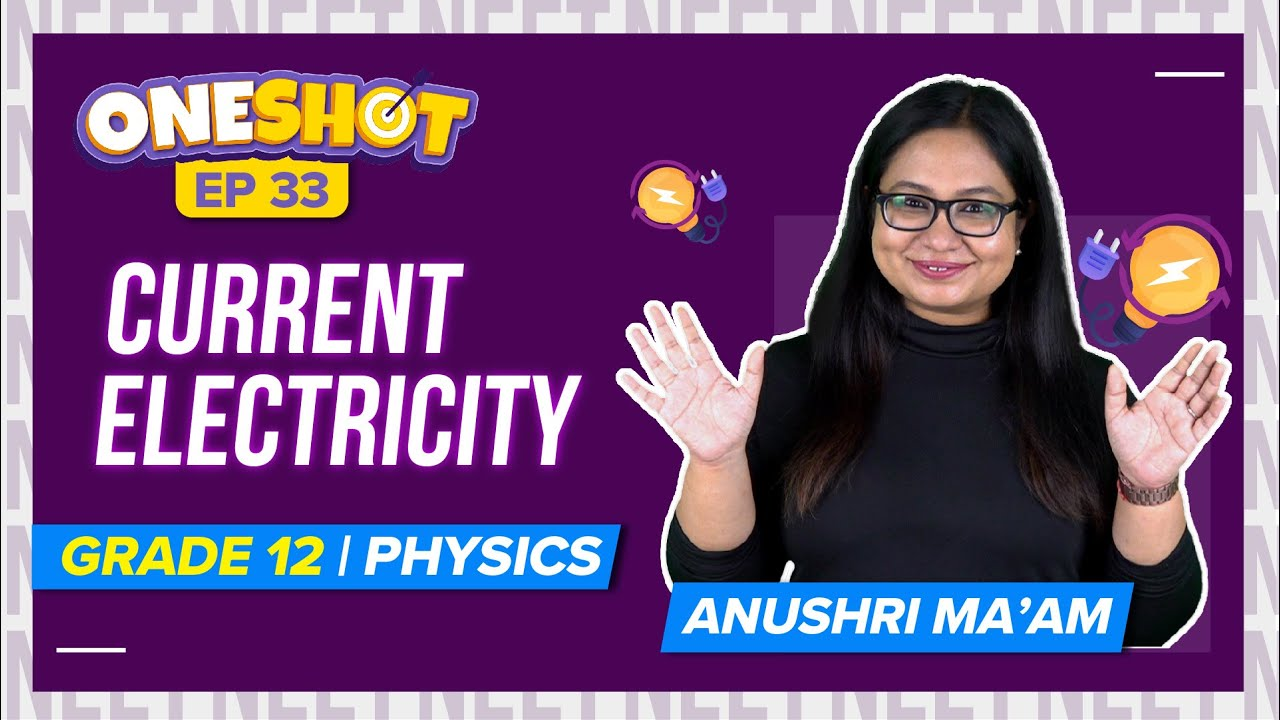Test your Knowledge on Exclusive OR Gate